Updating search results...

# 241 Results

View
Selected filters:
• fractions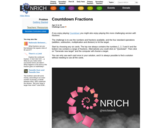Unrestricted Use
CC BY
Rating
0.0 stars

Countdown Fractions in this Nrich game offers a motivating context in which to practice calculating with fractions. There is usually more than one way of hitting the target, which offers an opportunity for rich discussion on the merits of alternative methods.

Subject:
Mathematics
Material Type:
Activity/Lab
Game
Interactive
06/17/2021Conditional Remix & Share Permitted
CC BY-NC-SA
Rating
0.0 stars

In this Cyberchase video segment, Shari Spotter gets help from the CyberSquad in doubling a recipe for crumpets.

Subject:
Mathematics
Material Type:
Lecture
Author:
U.S. Department of Education
WNET
Lynn Raith
08/04/2020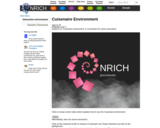Unrestricted Use
CC BY
Rating
0.0 stars

This Nrich interactive environment can be used with students, perhaps on an interactive whiteboard, for many different purposes. These might include, for example, exploring ideas associated with factors and multiples, or addition and subtraction. It is also an ideal context in which to investigate fractions and ratio, or to look at finding combinations.

Subject:
Mathematics
Material Type:
Activity/Lab
Interactive
06/17/2021Conditional Remix & Share Permitted
CC BY-NC-SA
Rating
0.0 stars

This short video and interactive assessment activity is designed to teach fifth graders about determining less than or greater than values of a decimal.

Subject:
Mathematics
Numbers and Operations
Material Type:
Assessment
Interactive
Lecture
Provider:
CK-12 Foundation
Provider Set:
CK-12 Elementary Math
04/03/2018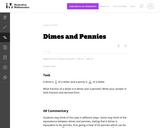Unrestricted Use
CC BY
Rating
0.0 stars

This task requires students to think of dimes and pennies as fractions of dollars.

Subject:
Mathematics
Numbers and Operations
Material Type:
Activity/Lab
Provider:
Illustrative Mathematics
Provider Set:
Illustrative Mathematics
Author:
Illustrative Mathematics
Institute for Mathematics & Education funded by the Bill & Melinda Gates Foundation
05/01/2012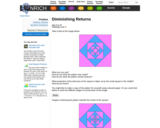Unrestricted Use
CC BY
Rating
0.0 stars

The Nrich problem offers opportunities to think about area, proportion and fractions, while offering an informal introduction to the mathematics of infinity and convergence which would not normally be met by younger students, to tempt their curiosity.

Subject:
Mathematics
Material Type:
Activity/Lab
06/17/2021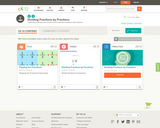Conditional Remix & Share Permitted
CC BY-NC-SA
Rating
0.0 stars

This short video and interactive assessment activity is designed to teach fifth graders about dividing fractions by fractions.

Subject:
Mathematics
Numbers and Operations
Material Type:
Assessment
Interactive
Lecture
Provider:
CK-12 Foundation
Provider Set:
CK-12 Elementary Math
04/03/2018Conditional Remix & Share Permitted
CC BY-NC-SA
Rating
0.0 stars

This short video and interactive assessment activity is designed to teach fifth graders about dividing whole numbers by fractions.

Subject:
Mathematics
Numbers and Operations
Material Type:
Assessment
Interactive
Lecture
Provider:
CK-12 Foundation
Provider Set:
CK-12 Elementary Math
04/03/2018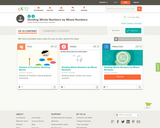Conditional Remix & Share Permitted
CC BY-NC-SA
Rating
0.0 stars

This short video and interactive assessment activity is designed to teach fifth graders about dividing whole numbers by mixed numbers.

Subject:
Mathematics
Numbers and Operations
Material Type:
Assessment
Interactive
Lecture
Provider:
CK-12 Foundation
Provider Set:
CK-12 Elementary Math
04/03/2018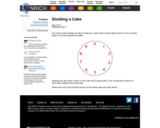Unrestricted Use
CC BY
Rating
0.0 stars

This Nrich problem gives learners practice in fractions (tenths) as well as in addition and subtraction in a challenging form.

Subject:
Mathematics
Material Type:
Activity/Lab
06/18/2021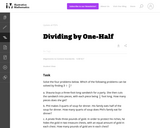Unrestricted Use
CC BY
Rating
0.0 stars

This task requires students to recognize both "number of groups unknown" and "group size unknown" division problems in the context of a whole number divided by a unit fraction.

Subject:
Mathematics
Numbers and Operations
Material Type:
Activity/Lab
Provider:
Illustrative Mathematics
Provider Set:
Illustrative Mathematics
Author:
Illustrative Mathematics
05/01/2012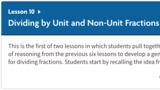Unrestricted Use
CC BY
Rating
0.0 stars

Unit 4: Dividing Fractions
Lesson 10: Dividing by Unit and Non-Unit Fractions

This is the first of two lessons in which students pull together the threads of reasoning from the previous six lessons to develop a general algorithm for dividing fractions. Students start by recalling the idea from grade 5 that dividing by a unit fraction has the same outcome as multiplying by the reciprocal of that unit fraction. They use tape diagrams to verify this.

Next, they use the same diagrams to look at the effects of dividing by non-unit fractions. Through repetition, they notice a pattern in the steps of their reasoning (MP8) and structure in the visual representation of these steps (MP7). Students see that division by a non-unit fraction can be thought of as having two steps: dividing by the unit fraction, and then dividing the result by the numerator of the fraction. In other words, to divide by 2/5 is equivalent to dividing by 1/5, and then again by 2. Because dividing by a unit fraction 1/5 is equivalent to multiplying by 5, we can evaluate division by 2/5 by multiplying by 5 and dividing by 2.

Subject:
Mathematics
Material Type:
Activity/Lab
04/14/2021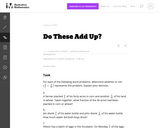Unrestricted Use
CC BY
Rating
0.0 stars

These word problems address common errors that students make when adding fractions.

Subject:
Mathematics
Numbers and Operations
Material Type:
Activity/Lab
Provider:
Illustrative Mathematics
Provider Set:
Illustrative Mathematics
Author:
Illustrative Mathematics
07/04/2012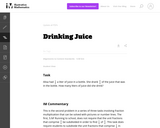Unrestricted Use
CC BY
Rating
0.0 stars

This problem involves fraction multiplication that can be solved with pictures or number lines.

Subject:
Mathematics
Numbers and Operations
Material Type:
Activity/Lab
Provider:
Illustrative Mathematics
Provider Set:
Illustrative Mathematics
Author:
Illustrative Mathematics
05/01/2012Conditional Remix & Share Permitted
CC BY-NC-SA
Rating
0.0 stars

In this activity , Harry gives an overview of working with fractions, decimals and percents, focusing on how to convert between them. Also included: the mini-game Stop the Pop, a comic video and quiz-show questions.

Subject:
Mathematics
Ratios and Proportions
Material Type:
Activity/Lab
Game
Interactive
08/04/2020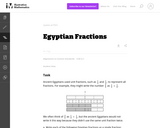Unrestricted Use
CC BY
Rating
0.0 stars

One goal of this task is to help students develop comfort and ease with adding fractions with unlike denominators. Another goal is to help them develop fraction number sense by having students decompose fractions. Because the Egyptians represented fractions differently than we do, it can also help students understand that there can be many ways of representing the same number.

Subject:
Mathematics
Numbers and Operations
Material Type:
Activity/Lab
Provider:
Illustrative Mathematics
Provider Set:
Illustrative Mathematics
Author:
Illustrative Mathematics
08/09/2012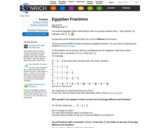Unrestricted Use
CC BY
Rating
0.0 stars

Unit fractions are the first fractions children meet, and here we discover some very surprising and interesting characteristics of these familiar numbers. Some of these characteristics were known to the ancient Egyptians whilst other conjectures are yet to be proved. Whilst meeting both old and new mathematical ideas, students can improve their fluency in addition and subtraction of fractions and be challenged to generalize and explain their findings.

Subject:
Mathematics
Material Type:
Activity/Lab
06/17/2021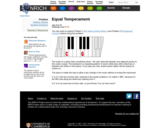Unrestricted Use
CC BY
Rating
0.0 stars

The topic of music can make a good connection between science and mathematics. The nature of sound and the working of the ear are rich areas of applied mathematics. The ratio emphasis follows from harmonics or overtones and rests on ideas like lowest common multiple.

Subject:
Mathematics
Material Type:
Activity/Lab
06/17/2021Rating
0.0 stars

The math learning center is an app and online platform that allows students to use manipulatives virtually. In this activity, students will show equivalent fractions using lines and shapes.

Subject:
Education
Educational Technology
Material Type:
Activity/Lab
Game
Interactive
Module
Provider:
REMC Association of Michigan
Author:
REMC Association of Michigan
03/13/2019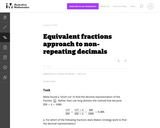Unrestricted Use
CC BY
Rating
0.0 stars

The purpose of the task is to get students to reflect on the definition of decimals as fractions (or sums of fractions), at a time when they are seeing them primarily as an extension of the base-ten number system and may have lost contact with the basic fraction meaning. Students also have their understanding of equivalent fractions and factors reinforced.

Subject:
Mathematics
Material Type:
Activity/Lab
Provider:
Illustrative Mathematics
Provider Set:
Illustrative Mathematics
Author:
Illustrative Mathematics